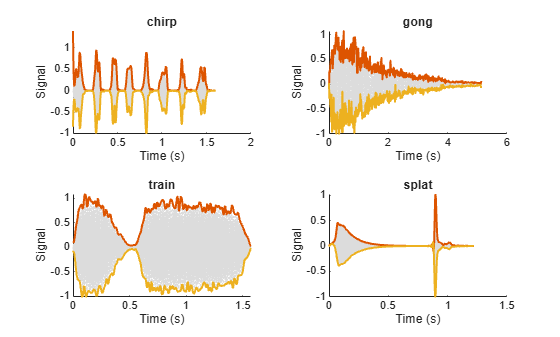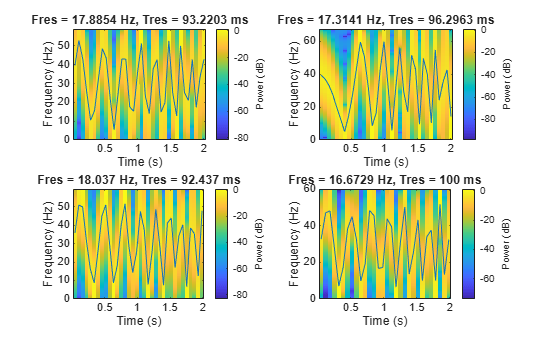combine

Combine data from multiple datastores

Description

example

sdsnew = combine(sds1,sds2,...,sdsn) combines two or more datastores by horizontally concatenating the data returned by the read function called on the input datastores.

Examples

collapse all

Specify the path to four signals included with MATLAB®. The signals are recordings of a bird chirp, a gong, a train, and a splat. All signals are sampled at 8192 Hz.

folder = fullfile(matlabroot,'toolbox','matlab','audiovideo', ...
["chirp.mat","gong.mat","train.mat","splat.mat"]);

Create a signal datastore that points to the specified files. Each file contains the variable Fs that denotes the sample rate.

sds1 = signalDatastore(folder,'SampleRateVariableName','Fs');

Define a function that takes the output of the read function and calculates the upper and lower envelopes of the signals using spline interpolation over local maxima separated by at least 80 samples. The function also returns the sample times for each signal.

function [dataOut,infoOut] = signalEnvelope(dataIn,info)
[dataOut(:,1),dataOut(:,2)] = envelope(dataIn,80,'peak');
infoOut = info;
infoOut.TimeInstants = (0:length(dataOut)-1)/info.SampleRate;
end

Call the transform function to create a second datastore, sds2, that computes the envelopes of the signals using the function you defined.

sds2 = transform(sds1,@signalEnvelope,"IncludeInfo",true);

Combine sds1 and sds2 create a third datastore. Each call to the read function from the combined datastore returns a matrix with three columns:

• The first column corresponds to the original signal.

• The second and third columns correspond to the upper and lower envelopes, respectively.

sdsCombined = combine(sds1,sds2);

Read and display the original data and the upper and lower envelopes from the combined datastore. Use the extractBetween function to extract the file name from the file path.

tiledlayout('flow')
while hasdata(sdsCombined)
ts = infoOut{2}.TimeInstants;
nexttile
hold on
plot(ts,dataOut(:,1),'Color','#DCDCDC','LineStyle',':')
plot(ts,dataOut(:,2:3),'Linewidth',1.5)
hold off
xlabel('Time (s)')
ylabel('Signal')
title(extractBetween(infoOut{:,2}.FileName,'audiovideo\','.mat'))
endSpecify the path to example files included with Signal Processing Toolbox™. Each file contains a chirp and a random sample rate ranging from 100 to 150 Hz.

folder = fullfile(matlabroot,'examples','signal','data','sample_chirps');

Create a signal datastore that points to the specified folder and set the names of the sample rate variables.

sds = signalDatastore(folder,'SampleRateVariableName','fs');

Define a function that takes the output of the read function and uses the pspectrum function to estimate the power spectrum of the signal. Use the estimate to compute the instantaneous frequency. The function also returns the vector of time instants corresponding to the centers of the windowed segments and the frequencies corresponding to the spectral estimates contained in the spectrograms of the signals.

function [dataOut,infoOut] = extractinstfreq(dataIn,info)
[P,F,T] = pspectrum(dataIn,info.SampleRate,'spectrogram',...
'TimeResolution',0.1,'OverlapPercent',40,'Leakage',0.8);
dataOut = {instfreq(P,F,T)'};
infoOut = info;
infoOut.CenterFrequencies = F;
infoOut.TimeInstants = T;
end

Call the transform function to create a new datastore that computes the instantaneous frequencies.

sds2 = transform(sds,@extractinstfreq,'IncludeInfo',true);

Because the data in sds2 is not horizontally concatenable with the data in sds, transform the data in sds into cell arrays.

sds1 = transform(sds,@(x) {x});

Combine sds1 and sds2. While the combined datastore has unread files, read from the new datastore and visualize the spectrograms. Overlay the instantaneous frequencies on the spectrograms.

sdsCombined = combine(sds1,sds2);
sdsSubset = subset(sdsCombined,[1,4,9,10]);
plotID = 1;
while hasdata(sdsSubset)
subplot(2,2,plotID)
pspectrum(sig{:,1},info{:,2}.SampleRate,'spectrogram', ...
'TimeResolution',0.1,'OverlapPercent',40,'Leakage',0.8)
hold on
plot(info{:,2}.TimeInstants',sig{:,2})
plotID = plotID + 1;
endInput Arguments

collapse all

Signal datastores to combine, specified as two or more comma-separated signalDatastore objects.

Output Arguments

collapse all

New datastore with the combined data, returned as a CombinedDatastore object.

Calling read on the combined datastore, horizontally concatenates the data by calling read on each input datastore.